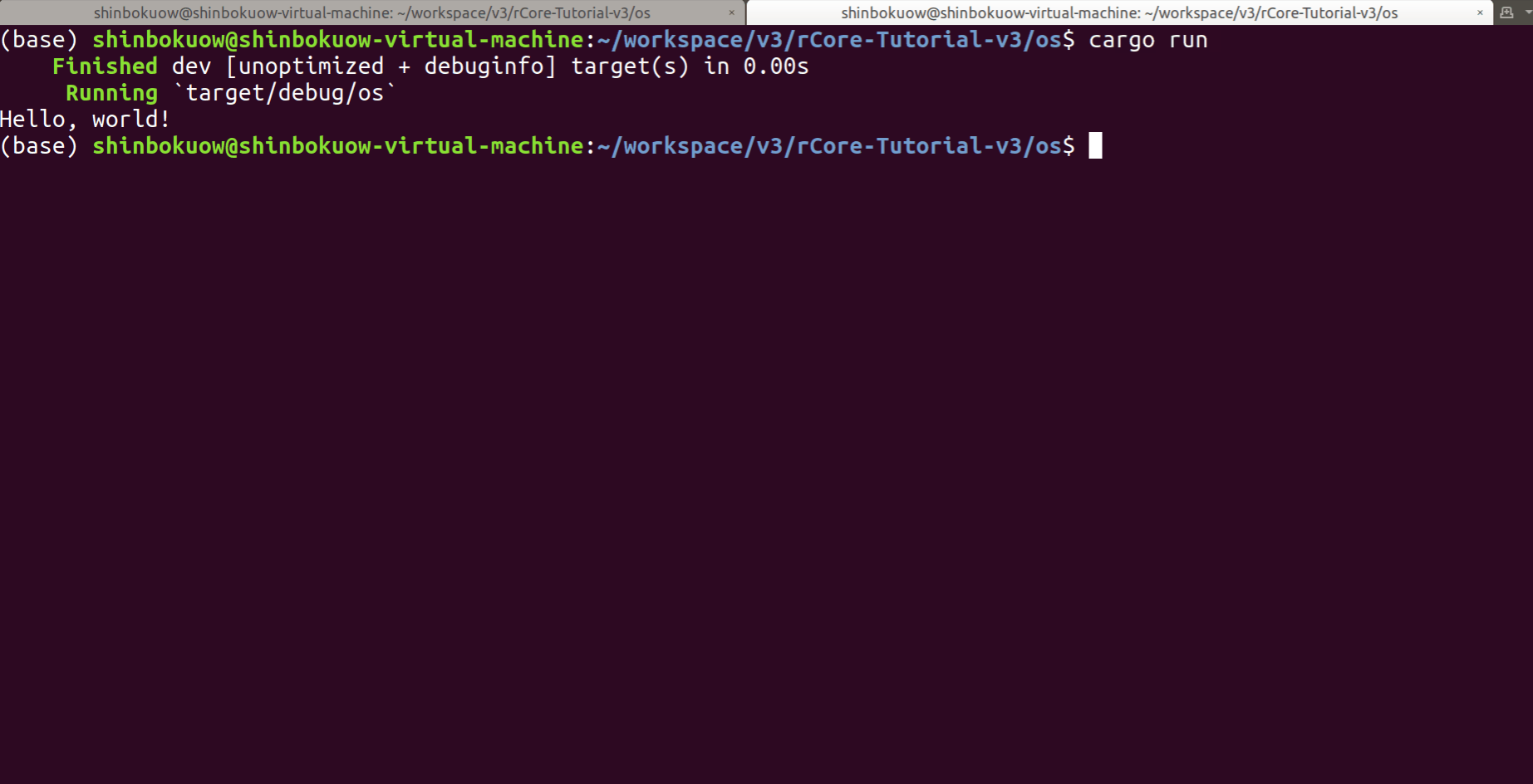# reStructuredText 基本语法#

可以使用 .. _process-exit: 记录文档的一个位置。



1// 我们甚至可以插入一段 Rust 代码！
2fn add(a: i32, b: i32) -> i32 { a + b }


Here is an attention.

it is dangerous!

here is a tip

this is important!

this is a hint.RISC-V 函数调用跳转指令#

$$\text{jal}\ \text{rd},\ \text{imm}[20:1]$$

$$\text{rd}\leftarrow\text{pc}+4$$

$$\text{pc}\leftarrow\text{pc}+\text{imm}$$

$$\text{jalr}\ \text{rd},\ (\text{imm}[11:0])\text{rs}$$

$$\text{rd}\leftarrow\text{pc}+4$$

$$\text{pc}\leftarrow\text{rs}+\text{imm}$$